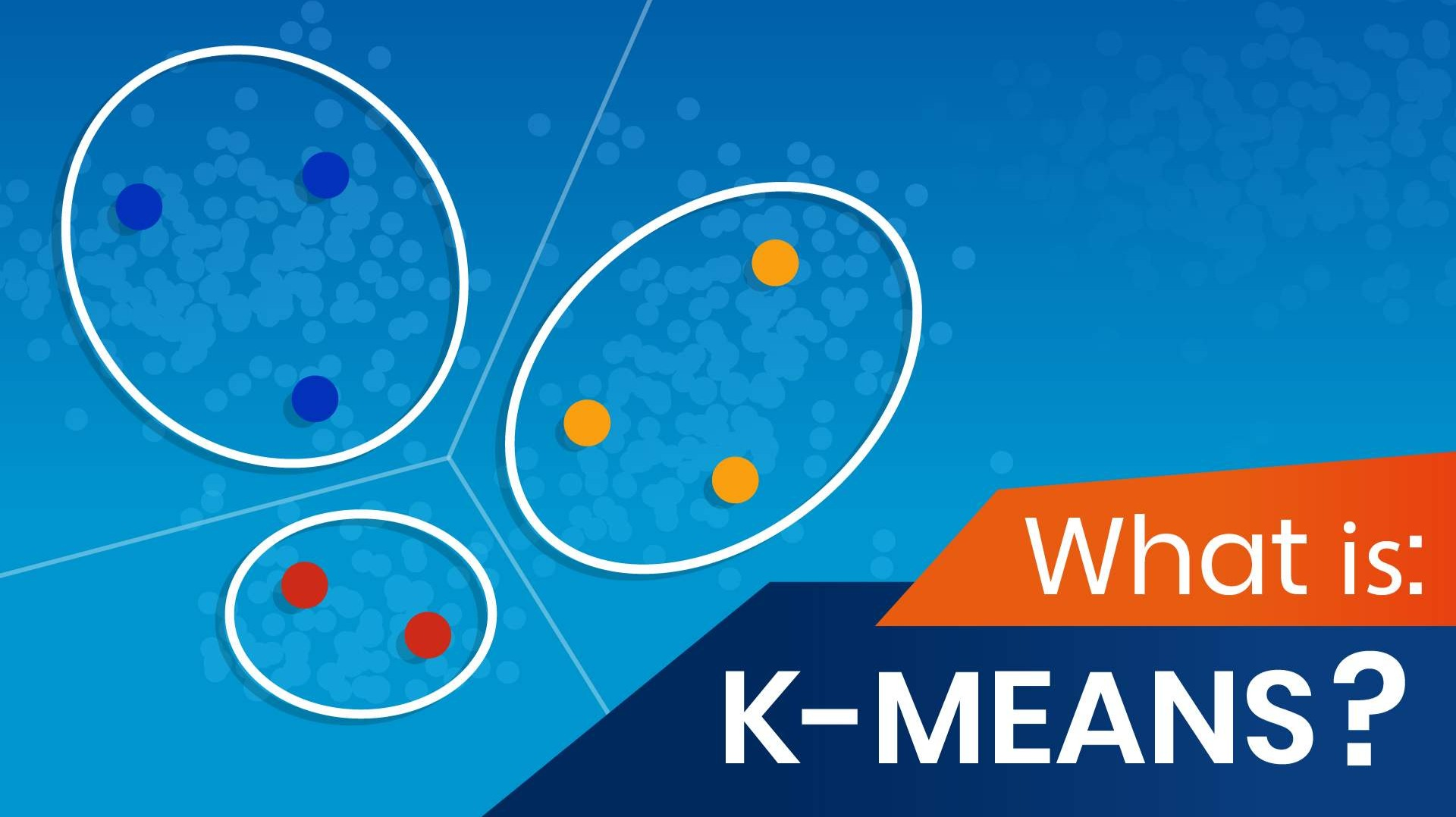# A brief Explanation of Machine Learning: K-Means Clustering

By eld3niz | A Brief Explanation | 27 Oct 2021

So far we have seen an example of the supervised learning technique K-Nearest-Neigbours. It was pretty straight-forward, but what if we want to use an unsupervised version in machine learning?

If you remember the general introduction, in supervised techniques we already have outputs resulting from our data so we can simply create a model which recommends possible outcomes for new given data. The exact opposite is the case when approaching unsupervised learning. K-Means Clustering is a great example of this so let's start!

Imagine you are given the task to separate some given points in a 2 dimensional space into fitting groups. With "dimensions" i am referring to the kind of label we are working with. These 2 labels can be either age & height or weight & health levels. There are infinite combinations not only in the categorical way, but only also in the amount of possible dimensions. Take a look at the following image with an arbitrary dimension 1 on the x-Axis and another dimension 2 on the y-Axis.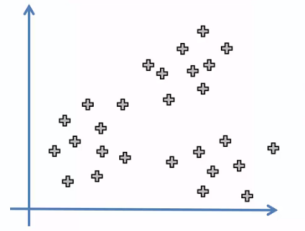You can choose in how many regions you want the dots to be divided into. Let's say you chose 3 regions. Pretty easy to show the result, right?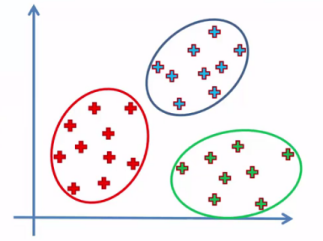If you haven't guessed the regions and circles right, don't worry. This is probably due to my lack of ability in explaining tasks. However real life data isn't as straight-forward as you have seen here. Altough there are many occasions where there are more than 2 dimensions, we will keep working with 2 (x1 and x2). Now here is another example, do the same thing: First choose a good number of regions and divide the dots into them in the most optimal way.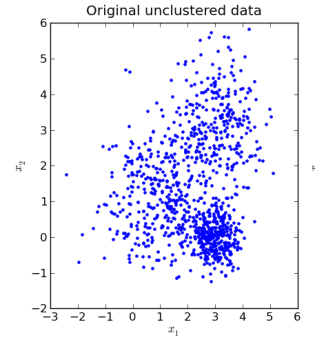Difficult, huh? Don't worry too much! That is the reason why we have tools like K-Means Clustering in our pocket. Before i show you guys the result, i will quickly explain how we can approach these kind of clustering problems through a very simple algorithm.

First of all, we want to have a measure of "how good" our clustering was. We will take the (for all math-phobic people skip this line) sum of squared distances from all the points to a given cluster-centre. The equation would take this form but this is not too important, just keep i mind, that, the bigger this number (sum of distances to the next centre), the worse our clustering is. This means we want to minimize J: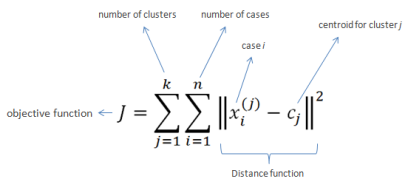How do we begin to place our centres of future regions. In our yet to solve image-cluster, we will place 3 dots (our cluster centres) randomly into the map. It really doesn't matter, where these are being placed. Now iterate through all of the given points on the map (of course except our new 3 centres) and decide to which of the 3 new points they are the nearest and mark it as a child of this point. Now every point in our space has 1 of the 3 new centres that is the nearest and the other 2 being further away. From now on i will call our new centres A, B and C. All the points being near to A might be still too far away. That is why we move A to centre of all A children in the following. Same approach with B, C and their children. When we have finished this process, begin the exact process again but with A, B and C now starting from there current positions, measuring distances from each point to A, B and C, deciding which of the three is the nearest, labeling as a child of A, B or C and  etc etc. After hopefully a few iterations of this we will see how we have found a Minimum J for A, B, and C in specific positions. This can look like this in the end: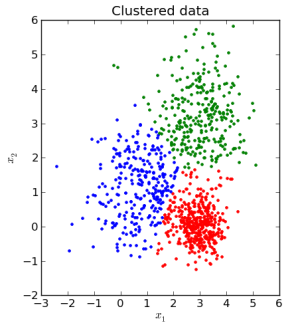Looks pretty cool right? Who tells us that this is the best approach? Well it is the math who tells us this the most optimal way. Here are the steps in short:

• Choose K and place them randomly on the map
• connect every original data point to its nearest K-centre
• place the K-centre into the middle of its connected points
• go to step 2 and repeat until the sum of points and K minimizes after a while

WAIT HOLD ON...... How do we know which K to choose???

This question is very interesting. I already mentioned how we can measure the "goodness" of our approach by considering the sum of squared distances J between K's and belonging data points. We can simply apply this algorithm with as many K's as possible and take a look with which amount of K's our results have the best outcome.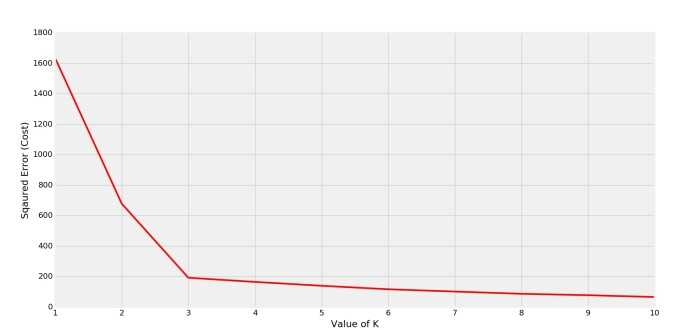I mean, trivially more K's will result in lower distances since every data point is its own centre. But when planning housepartys, you would never suggest everyone to stay at home and celebrate on their own to minimize their driving distance, right? That is a simplified reason why our K should be as low as possible. The image above display the so called Elbow-Approach to this problem. At K=3 we can clearly see how the error suddenly flattens. This is the K to choose. (L-formed error shape, hence Elbow).

This was an example of unsupervised-learning. Since you already know 2 techniques in machine learning you are kinda getting where this is heading- Yep right, a lot of math, trail and error. These topics can be very dry and exhausting but keep your head up, the following techniques will have some cool examples and open your eyes in how many of our real-life problems are being handled! Make sure to follow in order to not miss upcoming articles.

Puhhhh this was longer than i thought, but if you'd ask me what to take with you:

TL;DR: K-Means clustering tries to pack data points in different "regions" or clusters which can then be handled easier for given tasks. The number of clusters is given by amount of data points and their distance to the K-centres by a so called Elbow approach.eld3niz

Dont feel ashamed to ask. Looking forward to learn from everyone. Kinda interested in Blockchain and Physics. My goal is to describe complex things in the easiest way possible.A Brief Explanation

Welcome to the World of Physics, Math and Informatics! This blog will explain complex physical processes using simple examples from the microscopic to the macroscopic world.Send a \$0.01 microtip in crypto to the author, and earn yourself as you read!

20% to author / 80% to me.
We pay the tips from our rewards pool.